In a previous article, I showed how to use SAS to perform mean imputation. However, there are three problems with using mean-imputed variables in statistical analyses:

• Mean imputation reduces the variance of the imputed variables.
• Mean imputation shrinks standard errors, which invalidates most hypothesis tests and the calculation of confidence interval.
• Mean imputation does not preserve relationships between variables such as correlations.

This article explores these issues in more detail. The example data set (called IMPUTED) was created in the previous article. It is a modification of the Sashelp.Class data in which heights of seven students are assigned missing value. Mean imputation replaces those seven value with the mean of the observed values. The Orig_Height variable contains the original (missing) values; the Height variable contains the imputed values.

### Mean imputation reduces variance

The following call to PROC MEANS computes simple descriptive statistics for the original and imputed variables. The statistics for the original variable are computed by using listwise deletion, which means that missing observations are dropped from the analysis.

```proc means data=Imputed ndec=2 N NMiss Mean StdErr StdDev; var Orig_Height Height; /* compare stats original and imputed vars */ run;```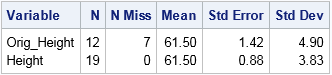The mean-imputed variable (Height) has the same mean as the original variable (Orig_Height). This is always the case for mean-imputed data. However, notice that the standard deviation (hence, variance) of the imputed variable is smaller. You can see this by overlaying the distributions of the original and imputed variables, as follows:

```title "Comparison of Original and Imputed Data"; proc sgplot data=Imputed; xaxis label="Height" values=(52 to 70 by 4 61.5) valueshint; yaxis grid; histogram Height / scale=count binstart=52 binwidth=4 legendlabel="Imputed"; histogram Orig_Height / scale=count binstart=52 binwidth=4 legendlabel="Original" transparency=0.5; refline 61.5 / axis=x lineattrs=(color=black) label="Mean"; /* mean value */ run;```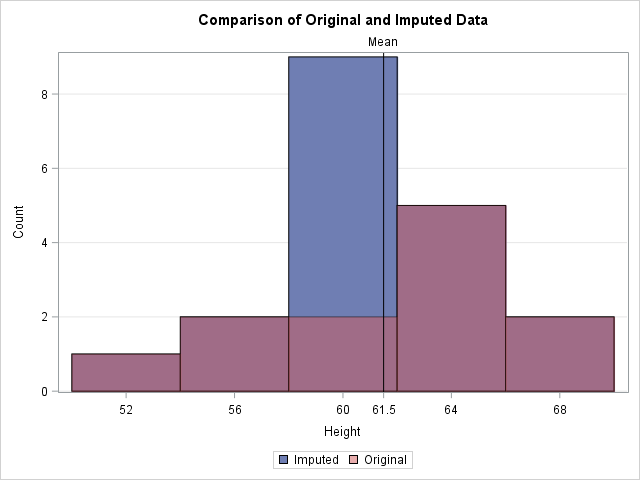In the graph, the reddish bars show the distribution of the observed values. Behind those bars is a second histogram (in blue) that shows the distribution of the imputed data. The only bar of the second histogram that is visible is the one that contains the sample mean. The graph emphasizes the fact that all imputed values are equal to the mean. This reduces the variance of the imputed variable because none of the imputed values contribute to the variance (which is based on deviations from the mean). Thus the variance of the mean-imputed variable is always smaller than the variance of the original variable.

### Mean imputation shrinks standard errors and confidence intervals

The previous section shows that the imputed variable always has a smaller variance than original variable. The estimated variance is used to compute many other statistics, which are also shrunk. For example, the following statistics are shrunk for the imputed variable as compared to the original variable:

1. The standard error of the mean, as shown in the previous output from PROC MEANS.
2. The confidence intervals that are based on mean-imputed data will be shorter.
3. The standard t test for a mean uses the standard error to compute a p-value for the null hypothesis that the population mean equals some value. If the standard error is shrunk by mean imputation, then the standard one-sample t test is not valid and the p-value is too small. You will potentially reject a null hypotheses that might be true (a Type I error).

### Mean imputation distorts relationships between variables

The previous sections emphasized how mean imputation affects univariate statistics. But mean imputation also distorts multivariate relationships and affects statistics such as correlation. For example, the following call to PROC CORR computes the correlation between the Orig_Height variable and the Weight and Age variables. It also computes the correlations for the mean-imputed variable (Height). The output shows that the imputed variable has much smaller correlations with Weight and Age because single imputation does not try to preserve multivariate relationships.

```proc corr data=Imputed noprob; var Weight Age; with Orig_Height Height; run;```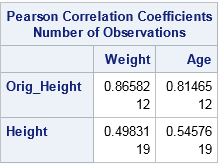In a similar way, a linear regression that attempts to predict Weight by height is corrupted by the replacement of missing values with mean values. For one-variable linear regression, it is easy to show that the estimates of the slope are unchanged by mean imputation, but the intercept estimates can be different. For these data, the least-squares estimate of the slope is 2.96. The intercept estimate for the original data is -90 whereas the intercept for the imputed variable is -82. The following call to PROC SGPLOT shows these estimates graphically:

```ods graphics / attrpriority=NONE; title "Simple Linear Regression with Mean Imputation"; proc sgplot data=Imputed; styleattrs datasymbols=(Circle X); reg x=Orig_Height y=Weight / nomarkers curvelabel="Original"; reg x=Height y=Weight / nomarkers curvelabel="Imputed"; scatter x=Height y=Weight / group=Replaced; xaxis grid; yaxis grid; run;```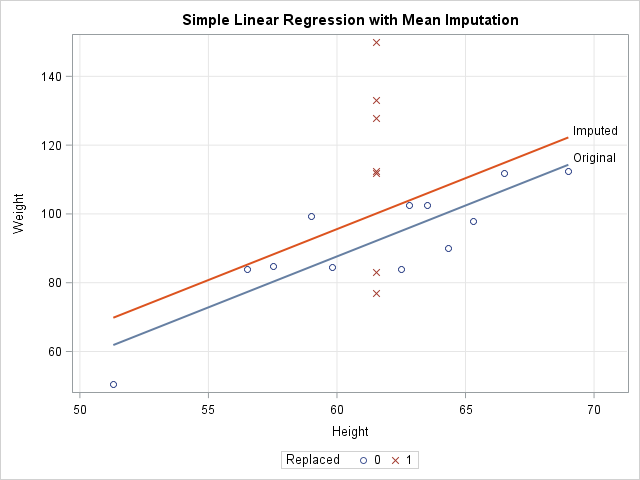The graph shows that the model that uses the original data (the blue line) predicts lower values of Weight than the model that uses the imputed heights (the red line). The scatter plot shows why. The model for the original data uses only 12 observations, which are displayed as blue circles. The predicted value of Weight for the mean height is about 92. The seven imputed values are shown as red X's for which the Height is 61.5. The average Weight for these observations is greater than 92, so the seven observations bias the computation and "pull up" the regression line. For different data, the imputed model might "pull down" the predictions. It depends on the average response for the imputed observations.

### Conclusions

Although imputing missing values by using the mean is a popular imputation technique, there are serious problems with mean imputation. The variance of a mean-imputed variable is always biased downward from the variance of the un-imputed variable. This bias affects standard errors, confidence intervals, and other inferential statistics. Experts agree that mean imputation should be avoided when possible (Allison (2009), Horton and Kleinman (2007)).

So what alternatives are there? That question has been the topic of many books and papers. Paul Allison (2009) suggests either maximum likelihood estimation or multiple imputation methods, both of which try to preserve relationships between variables and the inherent variability of the data. In SAS, PROC MI and MIANALYZE work with other SAS/STAT procedures to apply these methods to missing data. You can see the list of procedures that handle missing data in SAS. For more information about the alternatives to single imputation, the following references are good places to start:

The post 3 problems with mean imputation appeared first on The DO Loop.Imputing missing data is the act of replacing missing data by nonmissing values. Mean imputation replaces missing data in a numerical variable by the mean value of the nonmissing values. This article shows how to perform mean imputation in SAS. It also presents three statistical drawbacks of mean imputation.

### How to perform mean imputation in SAS

The easiest way to perform mean imputation in SAS is to use PROC STDIZE. PROC STDIZE supports the REPONLY and the METHOD=MEAN options, which tells it to replace missing values with the mean for the variables on the VAR statement. To demonstrate mean imputation, the following statements randomly add missing values to the Sashelp.Class data set. The call to PROC STDIZE then replaces the missing values and creates a data set called IMPUTED that contains the results:

```/* Create "original data" by randomly inserting missing values for some heights */ data Have; set sashelp.class; call streaminit(12345); Replaced = rand("Bernoulli", 0.4); /* indicator variable is 1 about 40% of time */ if Replaced then Height = .; run;   /* Mean imputation: Use PROC STDIZE to replace missing values with mean */ proc stdize data=Have out=Imputed oprefix=Orig_ /* prefix for original variables */ reponly /* only replace; do not standardize */ method=MEAN; /* or MEDIAN, MINIMUM, MIDRANGE, etc. */ var Height; /* you can list multiple variables to impute */ run;   proc print data=Imputed; format Orig_Height Height BESTD8.1; var Name Orig_Height Height Weight Replaced; run;```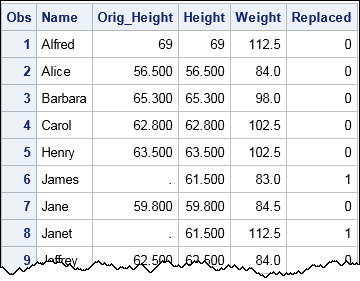The output shows that the missing data (such as observations 6 and 8) are replaced by 61.5, which is the mean value of the observed heights. For a subsequent visualization, I have included a binary variable (Replaced) that indicates whether an observation was originally missing. The METHOD= option in PROC STDIZE supports several statistics. You can use METHOD=MEDIAN to replace missing values by the median, METHOD=MINIMUM to replace by the minimum value, and so forth.

### Problems with mean imputation

Most software packages deal with missing data by using listwise deletion: observations that have missing data are dropped from the analysis. Throwing away hard-collected data is painful and can result in a substantial loss of power for statistical tests. Mean imputation, which is easy to implement, enables analysts to use every observation. However, mean imputation has three serious disadvantages that can lead to problems in your statistical analysis. Mean imputation is a univariate method that ignores the relationships between variables and makes no effort to represent the inherent variability in the data. In particular, when you replace missing data by a mean, you commit three statistical sins:

• Mean imputation reduces the variance of the imputed variables.
• Mean imputation shrinks standard errors, which invalidates most hypothesis tests and the calculation of confidence interval.
• Mean imputation does not preserve relationships between variables such as correlations.

These problems are discussed further in my next blog post. Most experts agree that the drawbacks far outweigh the advantages, especially since most software supports modern alternatives to single imputation, such as multiple imputation. My advice: don't use mean imputation if you can use a more sophisticated alternative.

### Epilogue

When I was in college, an actor friend smoked cigarettes. He knew that he should stop, but his addiction was too strong. When he lit up he would recite the following verse and dramatically punctuate the final phrase by blowing a smoke ring:

If you don't smoke, don't start.
If you do smoke, stop.
If you do smoke and won't stop, smoke with style. (*blows smoke ring*)

I don't recommend mean imputation. It is bad for the health of your data. But I can't dissuade from using mean imputation, remember the following verse:

If you don't use mean imputation, don't start.
If you do use mean imputation, stop.
If you do use mean imputation and won't stop, use PROC STDIZE.

The post Mean imputation in SAS appeared first on The DO Loop.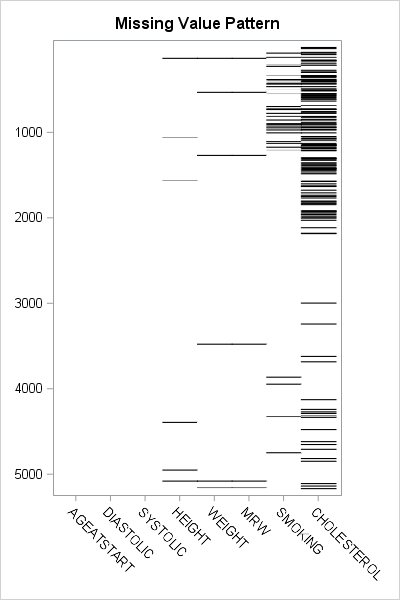Missing values present challenges for the statistical analyst and data scientist. Many modeling techniques (such as regression) exclude observations that contain missing values, which can reduce the sample size and reduce the power of a statistical analysis. Before you try to deal with missing values in an analysis (for example, by using multiple imputation), you need to understand which variables contain the missing values and to examine the patterns of missing values. I have previously written about how to use SAS to do the following:

An example of a visualization of a missing value pattern is shown to the right. The heat map shows the missing data for eight variables and 5209 observations in the Sashelp.Heart data set. It is essentially the heat map of a binary matrix where 1 indicates nonmissing values (white) and 0 indicates missing values (black).

In this article, I present a bar chart that helps to visualize the "Missing Data Patterns" table from PROC MI in SAS/STAT software. I also show two SAS tricks:

### The pattern of missing data

The MI procedure can perform multiple imputation of missing data, but it also can be used as a diagnostic tool to group observations according to the pattern of missing data. You can use the NIMPUTE=0 option to display the pattern of missing value. By default, the "Missing Data Patterns" table is very wide because it includes the group means for each variable. However, you can use the DISPLAYPATTERN=NOMEANS option (SAS9.4M5) to suppress the group means, as follows:

```%let Vars = AgeAtStart Height Weight Diastolic Systolic MRW Smoking Cholesterol; %let numVars = %sysfunc(countw(&Vars)); /* &numVars = 8 for this example */   proc mi data=Sashelp.Heart nimpute=0 displaypattern=nomeans; /* SAS 9.4M5 option */ var &Vars; ods output MissPattern=Pattern; /* output missing value pattern to data set */ run;```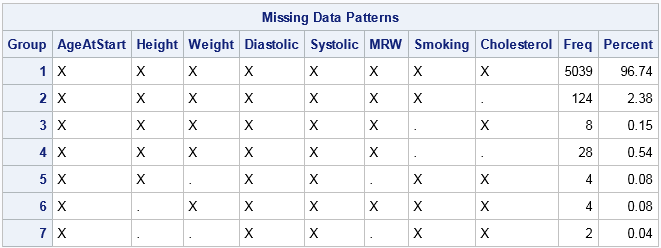In the table, an X indicates that a variable does not contain any missing values, whereas a dot (.) indicates that a variable contains missing values. The table shows that Group 1 consists of about 5000 observations that are complete. Groups 2, 3, and 6 contains observations for which exactly one variable contains missing values. Groups 4 and 5 contain observations for which two variables are jointly missing. Group 7 contains observations for which three variables are missing. You can see that the size of the groups vary widely. Some patterns of missing value appear only a few times, whereas others appear much more often.

By inspecting the table, you can determine which combinations of variables are missing for each group. However, imagine a dataset that has 20 variables. The "Missing Data Patterns" table for 20 variables would be very wide, and it would be cumbersome to determine which combination of variables are jointly missing. The next section condenses the table into a smaller format and then creates a graph to summarize the pattern of missing values.

### Shorten the labels for the missing data patterns

The information in the "Missing Data Patterns" table can be condensed. I saw the following idea in Chapter 10 of Gerhard Svolba's Data Quality for Analytics Using SAS, who credits the idea to a display that appears in JMP software. (Svolba does not use PROC MI, but defines a macro that you can download from the book's website.)

The table is wide because there is a column for every variable in the analysis. But the information in those columns is binary: for the i_th group does the j_th variable have a missing value? If the analysis involves k variables, you can replace the k columns by a single binary string that has k digits. The j_th digit in the string indicates whether the j_th variable has a missing value.

In the preceding analysis, the ODS OUTPUT statement wrote the "Missing Data Patterns" table to a data set. The following SAS DATA step reads the data and constructs a binary string of length k from the k character variables in the data. The binary string is formed by using the CATT function to concatenate the 'X' and '.' values in the table. The TRANSLATE function then converts those characters to '0' and '1' characters. The DATA step also computes the NumMiss variable, which counts the number of variables that have missing values for each row:

```data Miss; set Pattern; array vars[*] _CHARACTER_; /* or use &Vars */ length Pattern \$&numVars.; /* length = number of variables in array */ Pattern = translate(catt(of vars[*]), '01', 'X.'); /* Ex: 00100100 */ NumMiss = countc(pattern, '1'); run;   proc print data=Miss noobs; var Group Pattern NumMiss Freq; run;```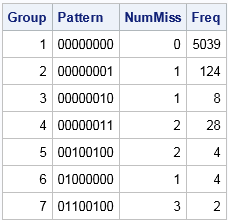The table shows that the eight columns for the analysis variables have been replaced by a single column that displays an eight-character binary string. For Group 1, the string is all zeros, which indicates that no variable has missing values. For Group 2, the binary string contains all zeros except for a 1 in the last position. This means that Group 1 is the set of observations for which the last variable contains a missing value. Similarly, Group 7 is the set of observations for which the second, third, and sixth variables contain a missing value. Notice that this table does not provide the names of the variables. If you cannot remember the name of the second, third, and sixth variables, you need to look them up in the VARS macro variable.

### Create a bar chart that has a logarithmic scale

The condensed version of the "Missing Data Patterns" table is suitable to graph as a bar chart. However, for these data the size of the groups vary widely. Consequently, you might want to plot the frequencies of the groups on a logarithmic scale. (Or you might not! There is considerable debate about whether you should display a bar chart that has a logarithmic axis. For a discussion and alternatives, see Sanjay's article "Graphs with log axis." My opinion is that a log axis is fine to use for a technical audience such as statisticians.)

By default, you cannot use a logarithmic scale on a bar chart because the baseline for the vertical axis (the frequency or count axis) starts at 0 and the LOG function is not defined at 0. If you have count data and no category has zero counts, then you can set the baseline of the graph to 1. The bars then indicate the log-frequency of the counts. The following call to PROC SGPLOT creates the bar chart and a marginal table:

```title "Pattern of Missing Values"; proc sgplot data=Miss; hbar Pattern /response=Freq datalabel baseline=1; /* set BASELINE=1 for log scale */ yaxistable NumMiss / valuejustify=left label="Num Miss" valueattrs=GraphValueText labelattrs=GraphLabelText; /* use same attributes as axis */ yaxis labelposition=top; xaxis grid type=log logbase=10 label="Frequency (log10 scale)"; run;```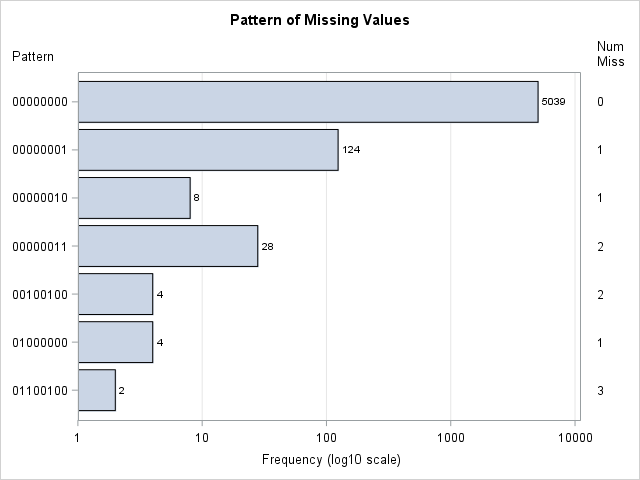This graph displays the counts of the number of observations in each pattern group. The labels on the Y axis indicate which variables have missing data. The values in the right margin indicate how many variables have missing data. If you are opposed to drawing bar charts on a logarithmic axis, you can use one of Sanjay's alternative visualizations.

In summary, you can create a bar chart that visualizes the number of observations that have a similar pattern of missing values. The summarization comes from PROC MI in SAS, which has a new DISPLAYPATTERN=NOMEANS option. A short DATA step can create a binary string for each group. That string can be used to indicate the missing data pattern in each group. If the groups vary widely in size, you can use a logarithmic axis to display the data. To create a bar chart on a logarithmic scale in SAS, set BASELINE=1 in the HBAR statement and use TYPE=LOG on the XAXIS statement in PROC SGPLOT.

The post Visualize patterns of missing values appeared first on The DO Loop.Missing values present challenges for the statistical analyst and data scientist. Many modeling techniques (such as regression) exclude observations that contain missing values, which can reduce the sample size and reduce the power of a statistical analysis. Before you try to deal with missing values in an analysis (for example, by using multiple imputation), you need to understand which variables contain the missing values and to examine the patterns of missing values. I have previously written about how to use SAS to do the following:

An example of a visualization of a missing value pattern is shown to the right. The heat map shows the missing data for eight variables and 5209 observations in the Sashelp.Heart data set. It is essentially the heat map of a binary matrix where 1 indicates nonmissing values (white) and 0 indicates missing values (black).

In this article, I present a bar chart that helps to visualize the "Missing Data Patterns" table from PROC MI in SAS/STAT software. I also show two SAS tricks:

### The pattern of missing data

The MI procedure can perform multiple imputation of missing data, but it also can be used as a diagnostic tool to group observations according to the pattern of missing data. You can use the NIMPUTE=0 option to display the pattern of missing value. By default, the "Missing Data Patterns" table is very wide because it includes the group means for each variable. However, you can use the DISPLAYPATTERN=NOMEANS option (SAS9.4M5) to suppress the group means, as follows:

```%let Vars = AgeAtStart Height Weight Diastolic Systolic MRW Smoking Cholesterol; %let numVars = %sysfunc(countw(&Vars)); /* &numVars = 8 for this example */   proc mi data=Sashelp.Heart nimpute=0 displaypattern=nomeans; /* SAS 9.4M5 option */ var &Vars; ods output MissPattern=Pattern; /* output missing value pattern to data set */ run;```In the table, an X indicates that a variable does not contain any missing values, whereas a dot (.) indicates that a variable contains missing values. The table shows that Group 1 consists of about 5000 observations that are complete. Groups 2, 3, and 6 contains observations for which exactly one variable contains missing values. Groups 4 and 5 contain observations for which two variables are jointly missing. Group 7 contains observations for which three variables are missing. You can see that the size of the groups vary widely. Some patterns of missing value appear only a few times, whereas others appear much more often.

By inspecting the table, you can determine which combinations of variables are missing for each group. However, imagine a dataset that has 20 variables. The "Missing Data Patterns" table for 20 variables would be very wide, and it would be cumbersome to determine which combination of variables are jointly missing. The next section condenses the table into a smaller format and then creates a graph to summarize the pattern of missing values.

### Shorten the labels for the missing data patterns

The information in the "Missing Data Patterns" table can be condensed. I saw the following idea in Chapter 10 of Gerhard Svolba's Data Quality for Analytics Using SAS, who credits the idea to a display that appears in JMP software. (Svolba does not use PROC MI, but defines a macro that you can download from the book's website.)

The table is wide because there is a column for every variable in the analysis. But the information in those columns is binary: for the i_th group does the j_th variable have a missing value? If the analysis involves k variables, you can replace the k columns by a single binary string that has k digits. The j_th digit in the string indicates whether the j_th variable has a missing value.

In the preceding analysis, the ODS OUTPUT statement wrote the "Missing Data Patterns" table to a data set. The following SAS DATA step reads the data and constructs a binary string of length k from the k character variables in the data. The binary string is formed by using the CATT function to concatenate the 'X' and '.' values in the table. The TRANSLATE function then converts those characters to '0' and '1' characters. The DATA step also computes the NumMiss variable, which counts the number of variables that have missing values for each row:

```data Miss; set Pattern; array vars[*] _CHARACTER_; /* or use &Vars */ length Pattern \$&numVars.; /* length = number of variables in array */ Pattern = translate(catt(of vars[*]), '01', 'X.'); /* Ex: 00100100 */ NumMiss = countc(pattern, '1'); run;   proc print data=Miss noobs; var Group Pattern NumMiss Freq; run;```The table shows that the eight columns for the analysis variables have been replaced by a single column that displays an eight-character binary string. For Group 1, the string is all zeros, which indicates that no variable has missing values. For Group 2, the binary string contains all zeros except for a 1 in the last position. This means that Group 1 is the set of observations for which the last variable contains a missing value. Similarly, Group 7 is the set of observations for which the second, third, and sixth variables contain a missing value. Notice that this table does not provide the names of the variables. If you cannot remember the name of the second, third, and sixth variables, you need to look them up in the VARS macro variable.

### Create a bar chart that has a logarithmic scale

The condensed version of the "Missing Data Patterns" table is suitable to graph as a bar chart. However, for these data the size of the groups vary widely. Consequently, you might want to plot the frequencies of the groups on a logarithmic scale. (Or you might not! There is considerable debate about whether you should display a bar chart that has a logarithmic axis. For a discussion and alternatives, see Sanjay's article "Graphs with log axis." My opinion is that a log axis is fine to use for a technical audience such as statisticians.)

By default, you cannot use a logarithmic scale on a bar chart because the baseline for the vertical axis (the frequency or count axis) starts at 0 and the LOG function is not defined at 0. If you have count data and no category has zero counts, then you can set the baseline of the graph to 1. The bars then indicate the log-frequency of the counts. The following call to PROC SGPLOT creates the bar chart and a marginal table:

```title "Pattern of Missing Values"; proc sgplot data=Miss; hbar Pattern /response=Freq datalabel baseline=1; /* set BASELINE=1 for log scale */ yaxistable NumMiss / valuejustify=left label="Num Miss" valueattrs=GraphValueText labelattrs=GraphLabelText; /* use same attributes as axis */ yaxis labelposition=top; xaxis grid type=log logbase=10 label="Frequency (log10 scale)"; run;```This graph displays the counts of the number of observations in each pattern group. The labels on the Y axis indicate which variables have missing data. The values in the right margin indicate how many variables have missing data. If you are opposed to drawing bar charts on a logarithmic axis, you can use one of Sanjay's alternative visualizations.

In summary, you can create a bar chart that visualizes the number of observations that have a similar pattern of missing values. The summarization comes from PROC MI in SAS, which has a new DISPLAYPATTERN=NOMEANS option. A short DATA step can create a binary string for each group. That string can be used to indicate the missing data pattern in each group. If the groups vary widely in size, you can use a logarithmic axis to display the data. To create a bar chart on a logarithmic scale in SAS, set BASELINE=1 in the HBAR statement and use TYPE=LOG on the XAXIS statement in PROC SGPLOT.

The post Visualize patterns of missing values appeared first on The DO Loop.When simulating data or testing algorithms, it is useful to be able to generate patterns of missing data. This article shows how to generate random and systematic patterns of missing values. In other words, this article shows how to replace nonmissing data with missing data.

Create patterns of missing data in #SAS
Click To Tweet

### Generate a random pattern of missing values

The following SAS/IML program reads numerical data into a matrix from the Sashelp.Class data set. The matrix has 16 rows and three columns. The program then generates a matrix of the same size that contains a random pattern of zeros and ones, where about 40% of the values will be ones. The LOC function is used to find the locations of the ones, and the corresponding locations in the data are set to missing:

```proc iml; use Sashelp.Class; /* read numeric data into X */ read all var _NUM_ into X; close;   /* random assignment of missing values */ RandX = X; /* copy data */ p = 0.4; /* approx proportion of missing elements */ call randseed(1234); B = randfun(dimension(X), "bern", p); /* random 0s or 1s */ missIdx = loc(B=1); /* find position of 1s */ if ncol(missIdx)>0 then RandX[missIdx] = .; /* replace 1s with missing */ print RandX;```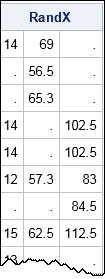In this way, you can replace a certain percentage of the data values with missing values.

### Generate a systematic pattern of missing values

In the preceding section, the technique for inserting missing values does not use the fact that the matrix B is random. The technique works with any zero-one matrix B that specifies a pattern of missing values. For example, you can create a matrix that contains all combinations of zeros and ones, then use that pattern to set missing values, as follows:

```C = { 0 0 0, 0 0 1, 0 1 0, 0 1 1, 1 0 0, 1 0 1, 1 1 0, 1 1 1 }; /* pattern matrix */   missIdx = loc(C=1); SysX = X; /* copy data */ if ncol(missIdx)>0 then SysX[missIdx] = .; /* replace 1s with missing */ print SysX;```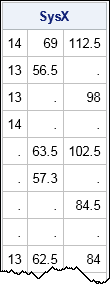You could also specify the locations of the missing values by using subscripts of the data matrix. You can use the SUB2NDX function to convert subscripts to indices.

### Patterns of missing data by using the SAS DATA step

In the SAS DATA step you can use arrays to create a random pattern of missing values. For example, the following SAS data set reads numerical variables from the Sashelp.Class data and randomly assigns 40% of the data to missing values:

```/* generate missing values in random locations */ data RandClass(drop=i); call streaminit(1234); set Sashelp.Class(keep=_NUMERIC_); array x {*} _numeric_; do i = 1 to dim(x); if rand("Bern", 0.4) then /* p=0.4 ==> about 40% missing */ x[i]=.; end; run;   proc print; run;```

The output is not shown, but the random pattern is identical to the random pattern that was generated by using SAS/IML matrices.

You could use the DATA step to specify patterns of missing values for which there is a formula, such as every fourth data value (MOD(cnt,4)=1). However, it is less easy to generate an arbitrary pattern, such as the "all combinations" pattern in the previous section. In general, I think the SAS/IML approach is easier to use and more flexible.

For any pattern of missing values, you can use PROC MI to summarize the pattern. You can also use various graphical techniques to visualize the pattern of missing data.

tags: Missing Data, Simulation

The post Create patterns of missing data appeared first on The DO Loop.You can visualize missing data. It sounds like an oxymoron, but it is true.

How can you draw graphs of something that is missing? In a previous article, I showed how you can use PROC MI in SAS/STAT software to create a table that shows patterns of missing data in SAS. In addition to tables, you can use graphics to visualize patterns of missing data.

It's not an oxymoron: Visualize missing data #Statistics #SAStip
Click To Tweet

### Counts of observations that contain missing values

As shown in the previous post, it is useful to be able to count missing values across rows as well as down columns. These row and column operations are easily accomplished in a matrix language such as SAS/IML. For example, the following SAS/IML statement read in data from the Sashelp.Heart data set. A single call to the COUNTMISS function counts the number of missing values in each row. The BAR subroutine creates a bar chart of the results:

```proc iml; varNames = {AgeAtStart Height Weight Diastolic Systolic MRW Smoking Cholesterol}; use Sashelp.Heart; /* open data set */ read all var varNames into X; /* create numeric data matrix, X */ close Sashelp.Heart;   title "Counts of Rows That Contain 0, 1, 2, or 3 Missing Values"; title2 "Sashelp.Heart Data"; Count = countmiss(X,"row"); /* count missing values for each obs */ call Bar(Count); /* create bar chart */```The bar chart clearly shows that most observations do not contain any missing values among the specified variables. A small percentage of observations contain one missing value. Even fewer contain two or three missing values.

### Which observations contain missing values?

It can be useful to visualize the locations of observations that contain missing values. Are missing values spread uniformly at random throughout the data? Or do missing values appear in clumps, which might indicate a systematic issue with the data collection?

The previous section computed the Count variable, which is a vector that has the same number of elements as there are rows in the data. To visualize the locations (rows) of missing values, use the LOC function to find the rows that have at least one missing value and plot the row indices:

```missRows = loc(Count > 0); /* which rows are missing? */ title "Location of Rows That Contain Missing Values"; call histogram( missRows ) scale="count" /* plot distribution */ rebin={125, 250} /* bin width = 250 */ label="Row Number"; /* label for X axis */```This histogram is very revealing. The bin width is 250, which means that each bar includes 250 observations. For the first 2000 observations, about 15 of every 250 observations contain a missing value. Then there is a series of about 500 observations that do not contain any missing observations. For the remaining observations, only about three of every 250 observations contain missing values. It appears that the prevalence of missing values changed after the first 2000 observations. Perhaps the patients in this data set were entered according to the date in which they entered the program. Perhaps after the first 2000 patients were recruited, there was a change in data collection that resulted in many fewer missing values.

### A heat map of the missing values

The ultimate visualization of missing data is to use a heat map to plot the entire data matrix. You can use one color (such as white) to represent nonmissing elements and another color (such as black) to represent missing values.

For many data sets (such as Sashelp.Heart), missing observations represent a small percentage of all rows. For data like that, the heat map is primarily white. Therefore, to save memory and computing time, it makes sense to visualize only the rows that have missing values. In the Sashelp.Heart data (which has 5209 rows), only 170 rows have missing values. For each of the 170 rows, you can plot which variables are missing.

The following statements implement this visualization of missing data in SAS. The matrix Y contains only the rows of the data matrix for which there is at least one missing value. You can call the CMISS function in Base SAS to obtain a binary matrix with values 0/1. The HEATMAPDISC subroutine in SAS/IML enables you to create a heat map of a matrix that has discrete values.

```ods graphics / width=400px height=600px; Y = X[missRows,]; /* extract missing rows */ call HeatmapDisc( cmiss(Y) ) /* CMISS returns 0/1 matrix */ displayoutlines=0 colorramp={white black} xvalues=VarNames /* variable names along bottom */ yvalues=missRows /* use nonmissing rows numbers as labels */ showlegend=0 title="Missing Value Pattern";```The black line segments represent missing values. This heat map summarizes almost all of the information about missing values in the data. You can see which variables have no missing values, which have a few, and which have many. You can see that the MRW variable is always missing when the Weight variable is missing. You can see that the first 2250 observations have relatively many missing values, that there is a set of observations that are complete, and that the missing values occur less frequently for the last 2000 rows.

If you do not have SAS/IML software, you can still create a discrete heat map by using the Graph Template Language (GTL).

Be aware that this heat map visualization is limited to data for which the number of rows that have missing values is somewhat small. (About 1000 rows or less.) In general, a heat map should contain at least one vertical pixel for each data row that you want to visualize. For the Sashelp.Heart data, there are 170 rows that have missing data, and the heat map in this example has 600 vertical pixels. If you have thousands of rows and try to construct a heat map that has only hundreds of pixels, then multiple data rows must be mapped onto a single row of pixels. The result is not defined, but certainly not optimal.

Do you ever visualize missing data in SAS? What techniques do you use? Has visualization revealed something about your data that you hadn't known before? Leave a comment.

The post Visualize missing data in SAS appeared first on The DO Loop.Missing data can be informative. Sometimes missing values in one variable are related to missing values in another variable. Other times missing values in one variable are independent of missing values in other variables. As part of the exploratory phase of data analysis, you should investigate whether there are patterns in the missing data.

Counting is the simplest analysis of any data (missing or otherwise). For each variable, how many observations are missing? You can then proceed to more complex analyses. Do two variable share a similar missing value pattern? Can you predict that one variable will be missing if you know that another variable is missing?

Missing data can be informative. Examine patterns of missing data #Statistics #SAStip
Click To Tweet

This article shows a simple way to examine patterns of missing values in SAS. The example data is the Sashelp.Heart data set, which contains information about patients in the Framingham Heart Study. The meaning of most variables is evident from the variable's name. An exception is the MRW variable, which contains a patient's "Metropolitan Relative Weight." The MRW is a percentage of the patient's weight to an ideal weight. Thus an MRW score of 100 means "ideal weight" whereas a score of 150 means "50% heavier than ideal." The MRW is similar to the more familiar body-mass index (BMI).

### The number of missing values for each variable

I have previously shown how to use PROC FREQ to count the number of missing values for numeric and character variables. The technique uses a custom format to classify each value as "Missing" or "Not Missing."

Alternatively, for numeric variables you can use PROC MEANS to count the number of missing values. PROC MEANS creates a compact easy-to-read table that summarizes the number of missing values for each numerical variable. The following statements use the N and NMISS options in the PROC MEANS statement to count the number of missing values in eight numerical variables in the Sashelp.Heart data set:

```/* count missing values for numeric variables */ proc means data=Sashelp.Heart nolabel N NMISS; var AgeAtStart Diastolic Systolic Height Weight MRW Smoking Cholesterol; run;```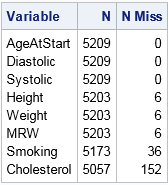The NMISS column in the table shows the number of missing values for each variable. There are 5209 observations in the data set. Three variables have zero missing values, and another three have six missing values. The Smoking variable has 36 missing values whereas the Cholesterol variable has 152 missing values.

These univariate counts are helpful, but they do not tell you whether missing values for different variables are related. For example, there are six missing values for the Height, Weight, and MRW variables. How many patients contributed to those six missing value? Ten? Twelve? Perhaps there are only six patients, each with missing values for all three variables? If a patient has a missing Height, does that imply that Weight or MRW is missing also? To answer these questions you must look at the pattern of missing values.

### Patterns of missing values

The MI procedure in SAS/STAT software is used for multiple imputation of missing values. PROC MI has an option to produce a table that summarizes the patterns of missing values among the observations. The following call to PROC MI uses the NIMPUTE=0 option to create the "Missing Data Patterns" table for the specified variables:

```ods select MissPattern; proc mi data=Sashelp.Heart nimpute=0; var AgeAtStart Height Weight Diastolic Systolic MRW Smoking Cholesterol; run;```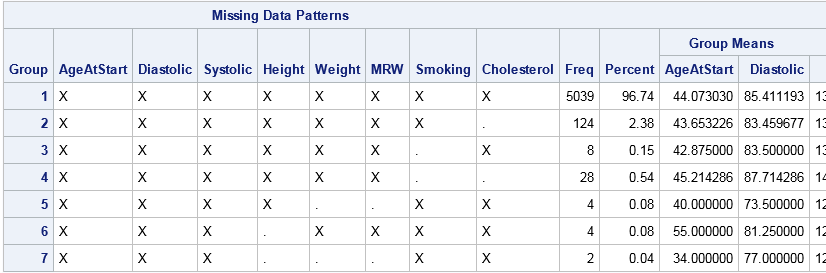The table reports the number of observations that have a common pattern of missing values. In addition to counts, the table reports mean values for each group. Because the table is very wide, I have truncated some of the mean values.

The first row counts the number of complete observation. There are 5039 observations that have no missing values.

Subsequent rows report the number of missing values for variables, pairs of variables, triplets of variables, and so forth. The variables are analyzed from right to left. Thus the second row shows that 124 observations have missing values for only the rightmost variable, which is Cholesterol. The third row shows that eight observations have missing values for only the Smoking variable. The fourth row shows that 28 observations have missing values for both Smoking and Cholesterol.

The table continues by analyzing the remaining variables that have missing values, which are Height, Weight, and MRW. You can see that MRW is never missing by itself, but that it is always missing when Weight is missing. Height is missing by itself in four cases, and is missing simultaneously with Weight in two cases.

Notice that each row of the table represents a disjoint set of observations. Consequently, we can easily answer the previous questions about how many patients contribute to the missing values in Height and Weight. There are 10 patients: four are missing only the Height measurement, four are missing only the Weight measurement (which forces MRW to be missing), and two are missing both Height and Weight.

This preliminary analysis has provided important information about the distribution of missing data in the Sashelp.Heart data set. Most patients (96.7%) have complete data. The most likely measurement to be missing is Cholesterol, followed by information about whether the patient smokes. You can see exactly how many patients are missing Height measurements, Weight measurements, or both. It is obvious that the MRW variable has a missing value if and only if the Weight variable is missing.

The "Missing Data Patterns" table from PROC MI provides a useful summary of missing values for each combination of variables. Examining patterns of missing values can lead to insight into the data collection process, and is also the first step prior to modeling missing data by using multiple imputation. In my next post, I will show how to use basic graphics to visualize patterns of missing data.

The post Examine patterns of missing data in SAS appeared first on The DO Loop.